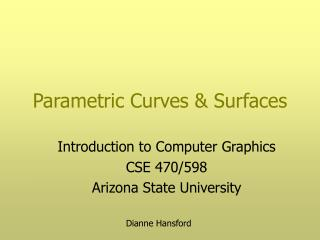Download PresentationParametric Curves & Surfaces

Loading in 2 Seconds...

# Parametric Curves & Surfaces - PowerPoint PPT Presentation

Parametric Curves & Surfaces. Introduction to Computer Graphics CSE 470/598 Arizona State University. Dianne Hansford. Overview. What is a parametric curve/surface? Why use parametric curves & surfaces? Bézier curves & surfaces NURBS Trimmed surfaces OpenGL library.I am the owner, or an agent authorized to act on behalf of the owner, of the copyrighted work described.
Download Presentation## Parametric Curves & Surfaces

An Image/Link below is provided (as is) to download presentation

Download Policy: Content on the Website is provided to you AS IS for your information and personal use and may not be sold / licensed / shared on other websites without getting consent from its author.While downloading, if for some reason you are not able to download a presentation, the publisher may have deleted the file from their server.

- - - - - - - - - - - - - - - - - - - - - - - - - - E N D - - - - - - - - - - - - - - - - - - - - - - - - - -
Presentation Transcript
1. Parametric Curves & Surfaces Introduction to Computer Graphics CSE 470/598 Arizona State University Dianne Hansford

2. Overview • What is a parametric curve/surface? • Why use parametric curves & surfaces? • Bézier curves & surfaces • NURBS • Trimmed surfaces • OpenGL library

3. What is a parametric curve? Recall functions from calculus ... Example: y = 2x – 2x2 To illustrate, we plot graph of function x 2x – 2x2 x y = Parametric curves give us more flexibility

4. What is a parametric curve? 2D parametric curve takes the form x y f(t) g(t) Where f(t) and g(t) are functions of t = Example: Line thru points a and b (1-t) ax + t bx (1-t) ay+ t by x y = Mapping of the real line to 2D: here t in [0,1]  line segment a,b

5. What is a parametric curve? 3D curves defined similarly x y z f(t) g(t) h(t) = Example: helix x y z cos(t) sin(t) t =

6. Bézier Curves Polynomial parametric curves f(t), g(t), h(t) are polynomial functions Bézier curve b(t) Bézier control points bi Bézier polygon Curve mimics shape of polygon t in [0,1] maps to curve “between” polygon b(0) = b0 and b(1) = bn figure: degree n=3 (cubic)

7. Bézier Curves Examples linear: b(t) = (1-t) b0 + t b1 n=1 quadratic: b(t) = (1-t)2b0 + 2(1-t)t b1 + t2b2 n=2 cubic: b(t) = (1-t)3b0 + 3(1-t)2 t b1 + 3(1-t)t2b2 + t3b3 n=3 Bernstein basis Bin (t) = {n!/(n-i)! i!} (1-t)n-i ti

8. Bézier Curves

9. Bézier Curves Bézier points and Bernstein basis • Nice, intuitive method to create curves • Variable display resolution • Minimal storage needs

10. Bézier Curves Bézier points and Bernstein basis nice, intuitive method to create curves Compare to Monomial basis: 1, t, t2, t3 ,.... ex: quadratic a(t) = a0 + t a1 + t2a2 a0 is point on curve a1 is first derivative vector a2 is second derivative vector Not very practical to design curves with! at t=0

11. Bézier Curves local and global parameter intervals Piecewise Bézier curves  global parameter u e.g., time Each curve evaluated for t in [0,1] [u0,u1] If specify u in global space then must find t in local space [u1,u2] t = (u-u0) / (u1-u0) figure: 2 quadratic curves

12. Bézier Curves Piecewise Bézier curves Conditions to create a smooth transition Filled squares are “junction” Bezier points-- start/endpoint of a curve

13. Bézier Curves in OGL Basic steps: • Define curve by specifying degree, control points and parameter space [u0,u1] • Enable evaluator • Call evaluator with parameter u in [u0, u1] glMap1*() Autocreate uniformly spaced u:glMapGrid1*() glEvalMesh1() Specify each u: glEvalCoord1*() or Color and texture available too!

14. Bézier Curve Evaluation de Casteljau algorithm another example of repeated subdivision On each polygon leg, construct a point in theratio t : (1-t) bn0(t) is point on curve figure: n=3

15. What is a parametric surface? 3D parametric surface takes the form Where f,g,h are bivariate functions of u and v x y z f(u,v) g(u,v) h(u,v) = Example: x(u,v) = u v u2 + v2 mapping u,v-space to 3-space; this happens to be a function too

16. Bézier Surface (Patch) Polynomial parametric surface f(u,v), g(u,v), h(u,v) are polynomial functions written in the Bernstein basis Bézier surface b(u,v) Bézier control points bij Bézier control net

17. Bézier Surface Structure b33 b03 (1,1) b30 v v u (0,0) b00 u

18. Bézier Surface Properties • boundary curves • lie on surface • boundary curves • defined by • boundary polygons

19. Bézier Surface Properties • Nice, intuitive method for creating surfaces • Variable display resolution • Minimal storage

20. Bézier Surface Multiple patches connected smoothly Conditions on control netsimilar to curves … difficult to do manually

21. Bézier Surface Display shaded wireframe OGL: triangles & normals created for you choose direction isoparametric curves OGL:glMap2*, glEvalCoord2* glMapGrid2, glEvalMesh2

22. NURBS Non-uniform Rational B-splines B-splines are piecewise polynomials One or more Bezier curves /surfaces One control polygon Rational: let’s us represent circles exactly GLU NURBS utility

23. Trimmed Surfaces Parametric surface with parts of the domain “invisible” Surf Lab domain Jorg Peters’ UFL group GLU Trimmed NURBS utility

24. References • The Essentials of CAGDby Gerald Farin & DCH, AK Petershttp://eros.cagd.eas.asu.edu/%7Efarin/essbook/essbook.html • Ken Joy’s CAGD notes (UC Davis)http://graphics.cs.ucdavis.edu/CAGDNotes/homepage.html • Jorg Peters’ UFL SurfLab grouphttp://www.cise.ufl.edu/research/SurfLab/index.html • OpenGL Red Book – Chapter 12